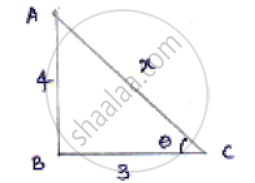Share

# If Cot Theta = 3/4 Prove that Sqrt((Sec Theta - Cosec Theta)/(Sec Theta +Cosec Theta)) = 1/Sqrt7 - CBSE Class 10 - Mathematics

#### Question

if cot theta = 3/4 prove that sqrt((sec theta - cosec theta)/(sec theta +cosec theta)) = 1/sqrt7

#### Solution

cot theta = "𝑎𝑑𝑗𝑎𝑐𝑒𝑛𝑡 𝑠𝑖𝑑𝑒"/"𝑜𝑝𝑝𝑜𝑠𝑖𝑡𝑒 𝑠𝑖𝑑𝑒"Let x be the hypotenuse by applying Pythagoras theorem.

𝐴𝐶2 = 𝐴𝐵2 + 𝐵𝐶2

𝑥2 = 16 + 9

x^2 = 25 => x = 5

sec theta = (AC)/(AB) = 5/4

cosec theta = (AC)/(AB) = 5/4

On substituting in equation we get

sqrt((sec theta - cosec theta)/(sec theta + cosec theta)) = sqrt((5/3 - 5/4)/(5/3 + 5/4))

= sqrt(((20 - 15)/12)/((20 + 15)/12)) = sqrt(5/35) = 1/sqrt7

Is there an error in this question or solution?

#### APPEARS IN

Solution If Cot Theta = 3/4 Prove that Sqrt((Sec Theta - Cosec Theta)/(Sec Theta +Cosec Theta)) = 1/Sqrt7 Concept: Trigonometric Ratios.
S